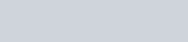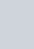Five cards−ten, jack, queen, king, and an ace of diamonds
Question:

Five cards−ten, jack, queen, king, and an ace of diamonds are shuffled face downwards. One card is picked at random.

(i) What is the probability that the card is a queen?

(ii) If a king is drawn first and put aside, what is the probability that the second card picked up is the ace?

Solution:

GIVEN: Five cards-ten, jack, queen, king and Ace of diamond are shuffled face downwards

TO FIND: Probability of following

Total number of cards are5

(i) Cards which is a queen

Total number of Cards which are queen is 1

Number of favorable event i.e. Total number of Cards which are queen is 1

We know that PROBABILITY =Hence probability of getting cards which are queen(ii) If a king is drawn first and put aside then

Total number of cards is 4

Number of favorable event i.e. Total number of ace card is 1

We know that PROBABILITY =Hence probability of getting ace cards